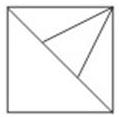Question 2

# How many triangles are there in the given figure ?Solution

Small triangles = ABE, BEF, BFD, ACD

Triangles (having 2 triangles) = ABF, BED

Triangles (having 3 triangles) = ABD

Thus, total triangles = 7

=> Ans - (C)